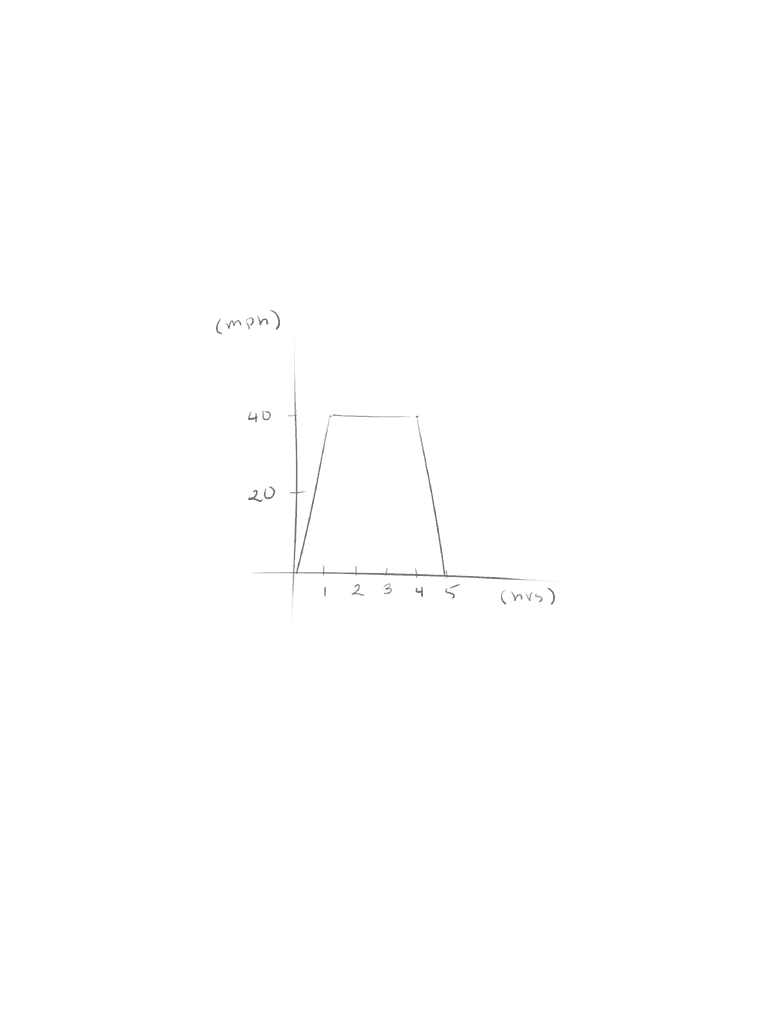# MTH 151 Midterm: MTH 151 - Term Test 3

140 views25 pages
Miami University
MTH 151
Calculus I
Winter 2018
Term Test 3
Prof: Olga Brezhneva
Exam Guide
Unlock document

This preview shows pages 1-3 of the document.
Unlock all 25 pages and 3 million more documents.CONTENTS
Exam Guide Covers the Following Topics:
Unit 3: Chapter 5: Integrals
Areas and Distances
Definite Integral
The Fundamental Theorem of Calculus
Indefinite Integrals and the Net Change Theorem
Substitution
Unlock document

This preview shows pages 1-3 of the document.
Unlock all 25 pages and 3 million more documents.Areas and Distances
Typically seen questions
Given the graph, what is the distance travelled
Between 1 and 4 pm?
In total? (from t = 0 to t = 5)
So we know that the graph shows the relationship between velocity (miles per hour)
and time (hours)
Because D (distance) is equal to the product of R (rate) and T (time), we can find
and solve for distance using the formula D = RT
Using the givens, between 1 and 4 pm is 3 hours, and the speed is constant at 40
mph
So D = (40)(3) = 120 miles
Looking at the graph, you may realize that 120 is equal to the area of the rectangle
formed using the time as a base and velocity as a height
Unlock document

This preview shows pages 1-3 of the document.
Unlock all 25 pages and 3 million more documents.

## Document Summary

Given the graph, what is the distance travelled . In total? (from t = 0 to t = 5) So we know that the graph shows the relationship between velocity (miles per hour) and time (hours) Using the givens, between 1 and 4 pm is 3 hours, and the speed is constant at 40 mph. So d = (40)(3) = 120 miles. This is a fact that within a velocity time graph, the area under the curve is the distance travelled. Knowing this information, we can then solve for the distance by finding the area under the curve between t = 0 and t = 5. We can either break this into two triangles and a rectangle, or simply use the equation of area for a trapezoid. ( )(b1 + b2)(h) in which b1 is equal to base 1, b2 is base 2, and h is the height. So say we have the function y = x^2.